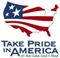Scientific Investigations Report 2009–5015

U.S. GEOLOGICAL SURVEY
Scientific Investigations Report 2009–5015

Table 1. Regression equations for the low-flow statistic, 7Q2 , for unregulated streams in regions 1–8, Idaho.

[Locations of regions are shown in figure 1. Standard error of prediction: Values determined using error transformation equations in Riggs (1968). Abbreviations: 7Q2, 7-day 2-year low flow, in cubic feet per second; A, drainage area, in square miles; BS, basin slope, in percent; E, mean elevation, in feet; P, mean annual precipitation, in inches; R, basin relief, in feet; S50, slopes greater than 50 percent in percentage of drainage area; V, surficial volcanic rocks, in percentage of drainage area; W, water in percentage of drainage area]

Region 7Q2 regression equation Standard error of prediction
Original (Hortness, 2006) Revised log10 Percent
1 7Q2 = 0.731A0.613(W+1)0.907 7Q2 = 0.300A0.879 0.271 +86.7 to -46.4
12 7Q2 = 0.000153A1.04P1.92 No change 0.253 +78.9 to -44.1
3 7Q2 = 0.00953A0.392(R/1,000)3.36 7Q2 = 0.0214A0.971 0.277 +236 to -70.2
14 7Q2 = 0.0000215A1.04P2.41 No change 0.341 +119 to -54.4
5 7Q2 = 0.000453A1.04(V+1)1.51 7Q2 = 0.334A0.963 0.249 +77.3 to -43.6
16 7Q2 = 0.000133A1.05P2.10 No change 0.243 +75.0 to -42.9
7 7Q2 = 0.0329A0.678(S50+1)0.796 7Q2 = 0.0000115A0.837(E/1000)4.658 0.577 +277 to -73.5
8 7Q2 = 3.86A0.930BS-0.648 7Q2 = 0.00181A0.981P1.515 0.331 +116 to -53.4

1Denotes region with original Hortness (2006) equation.U.S. Department of the Interior | U.S. Geological Survey